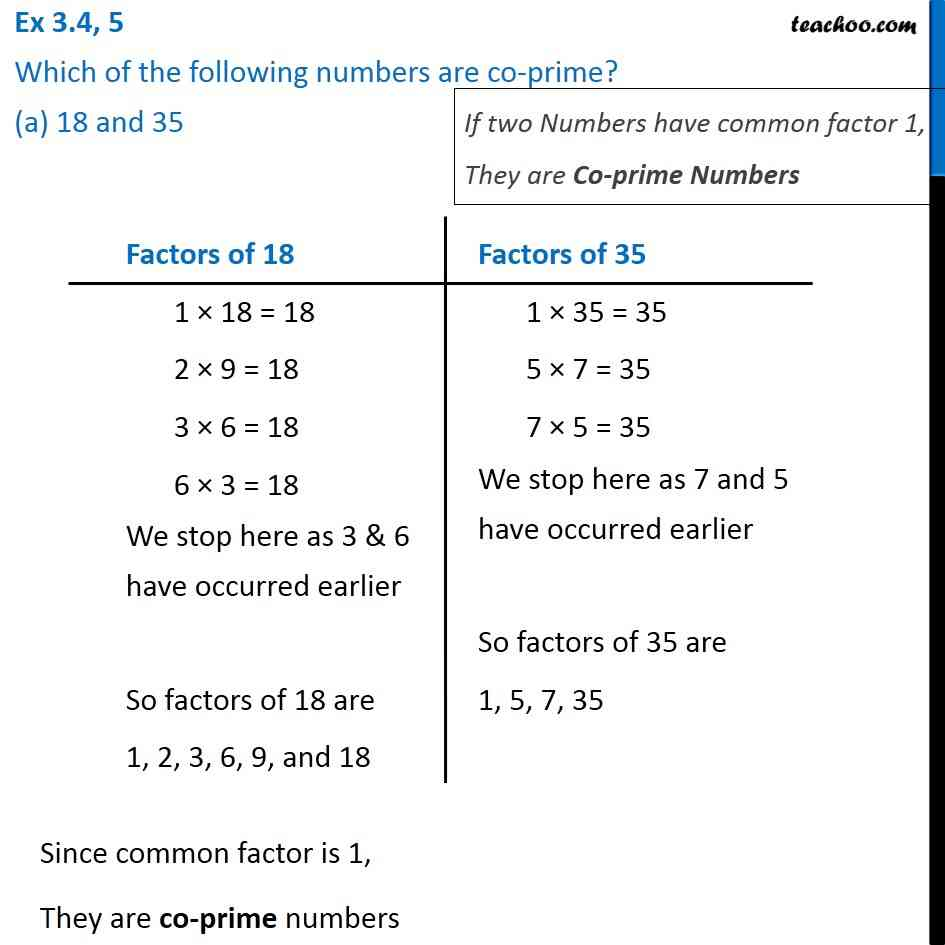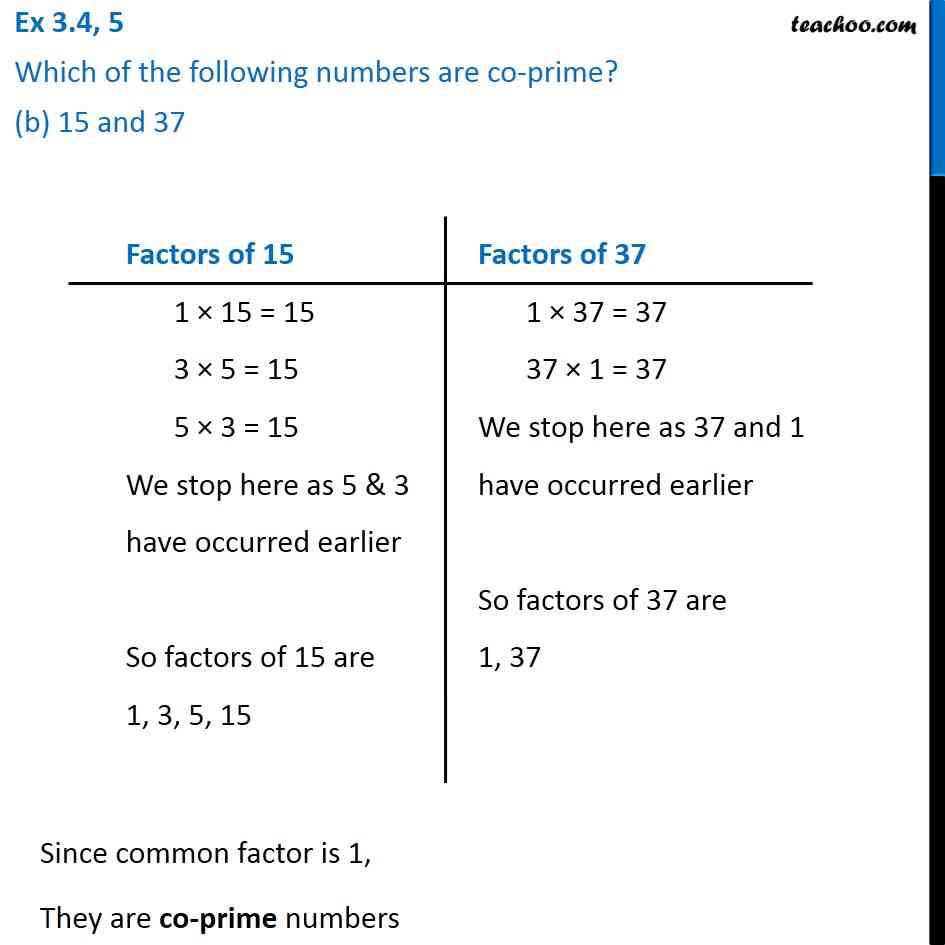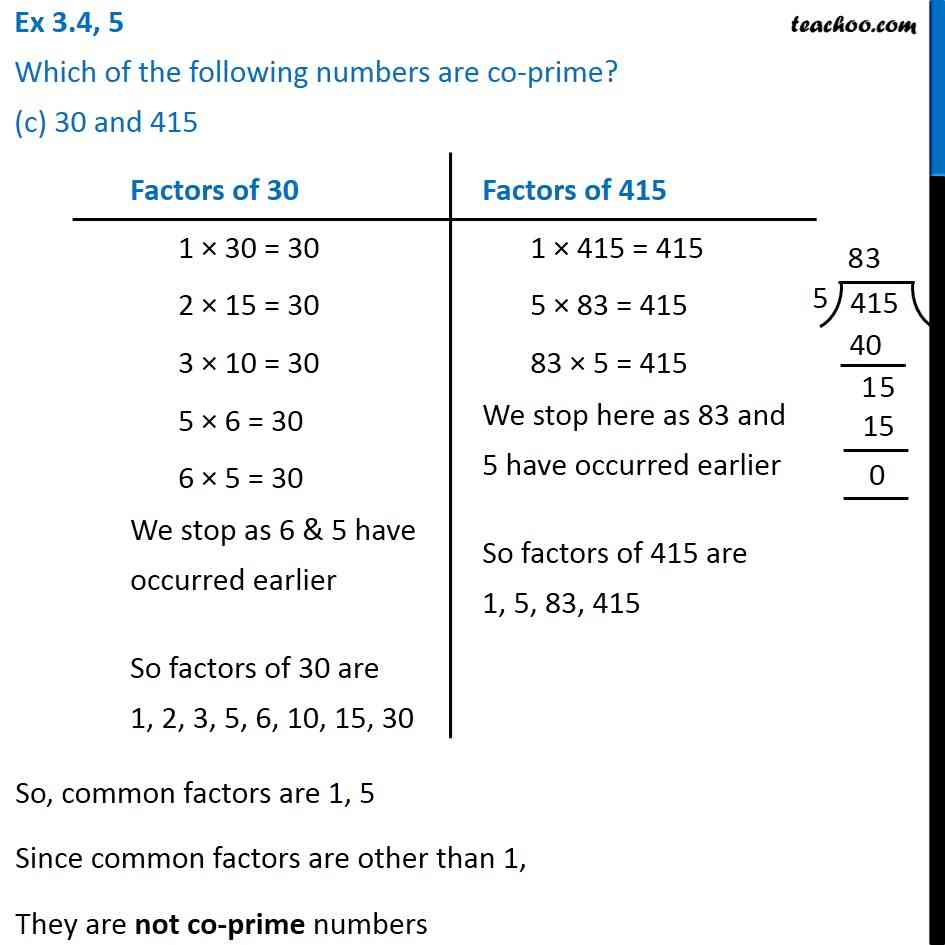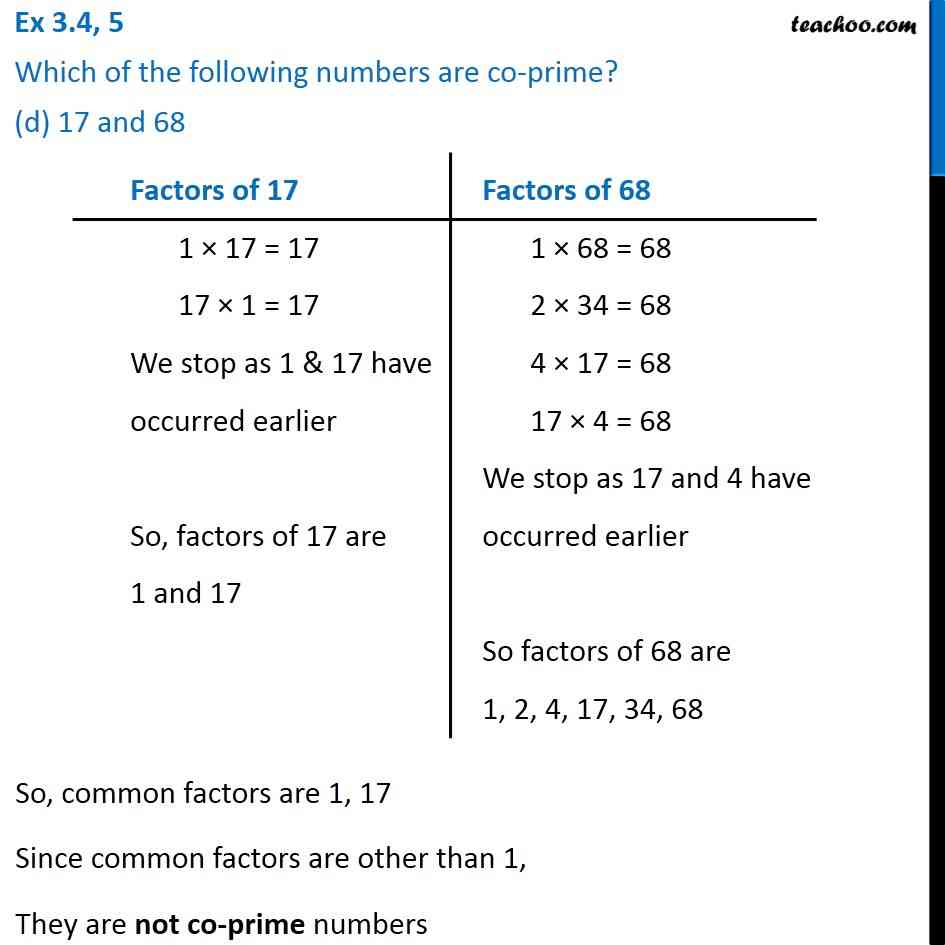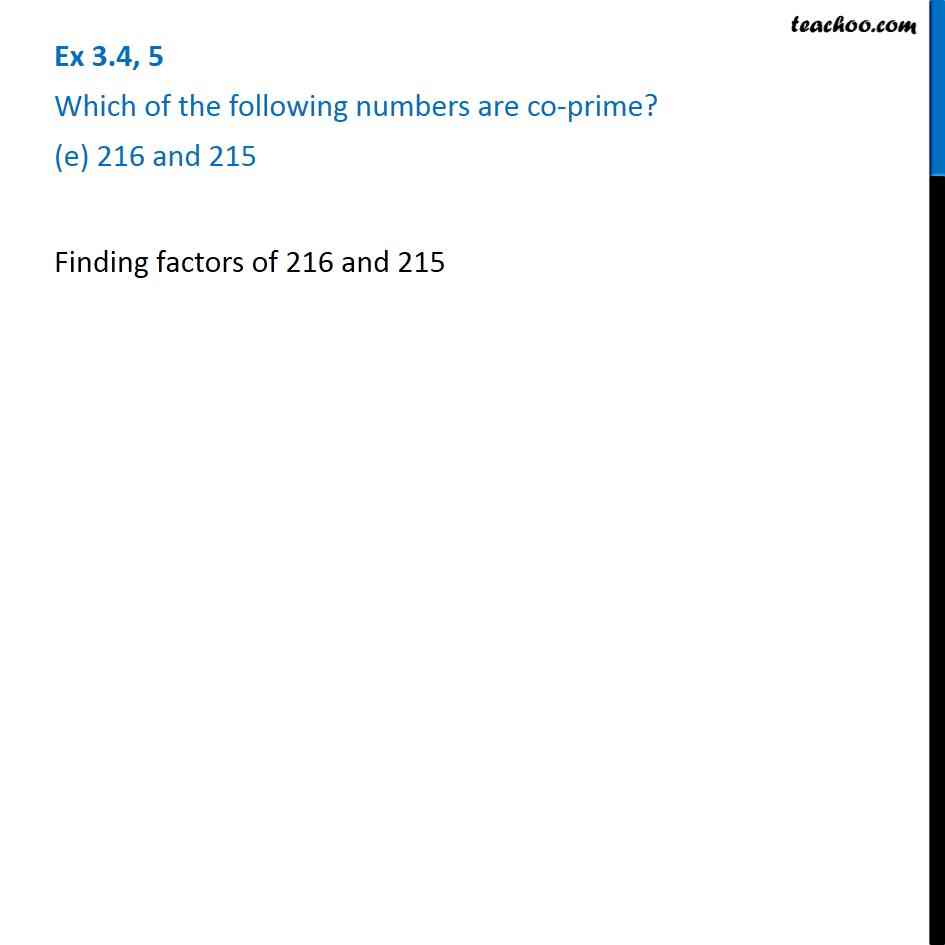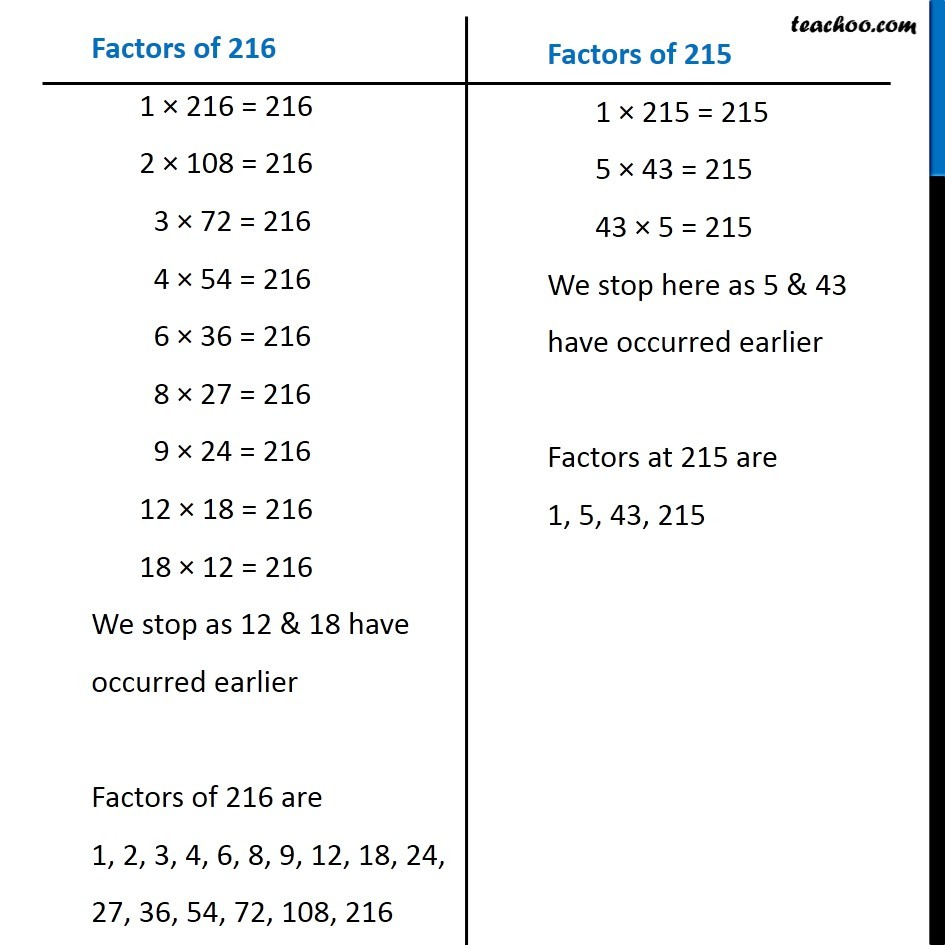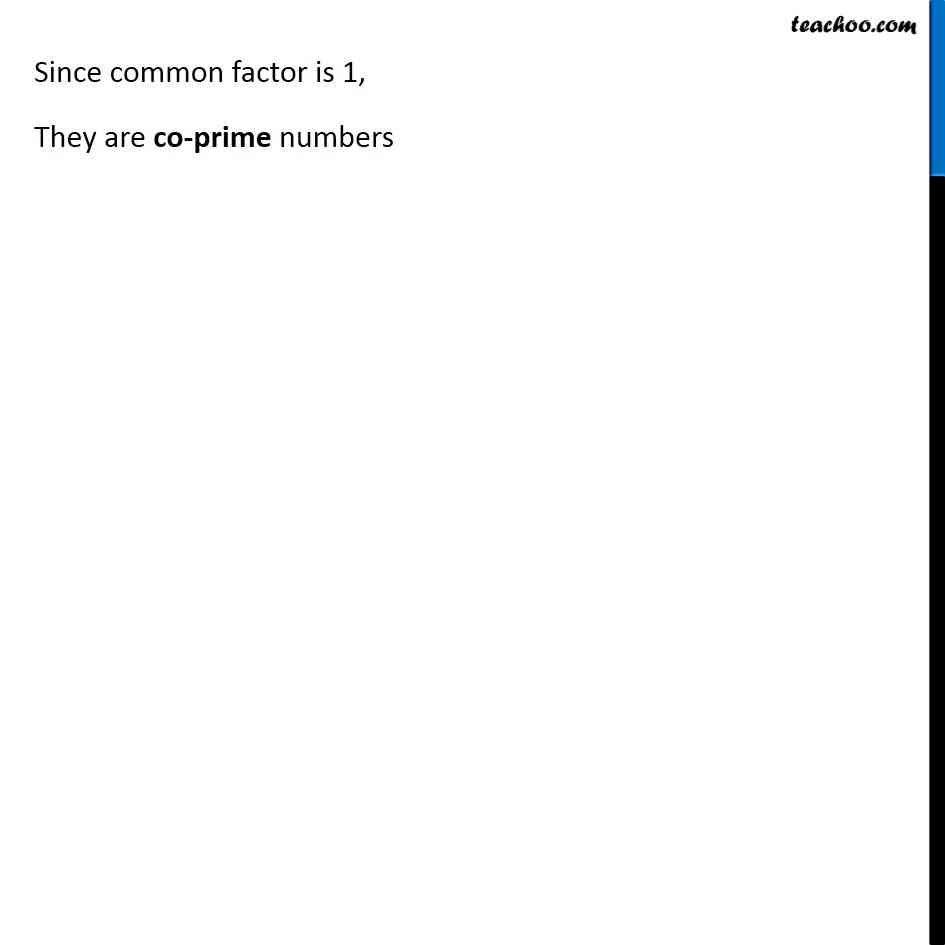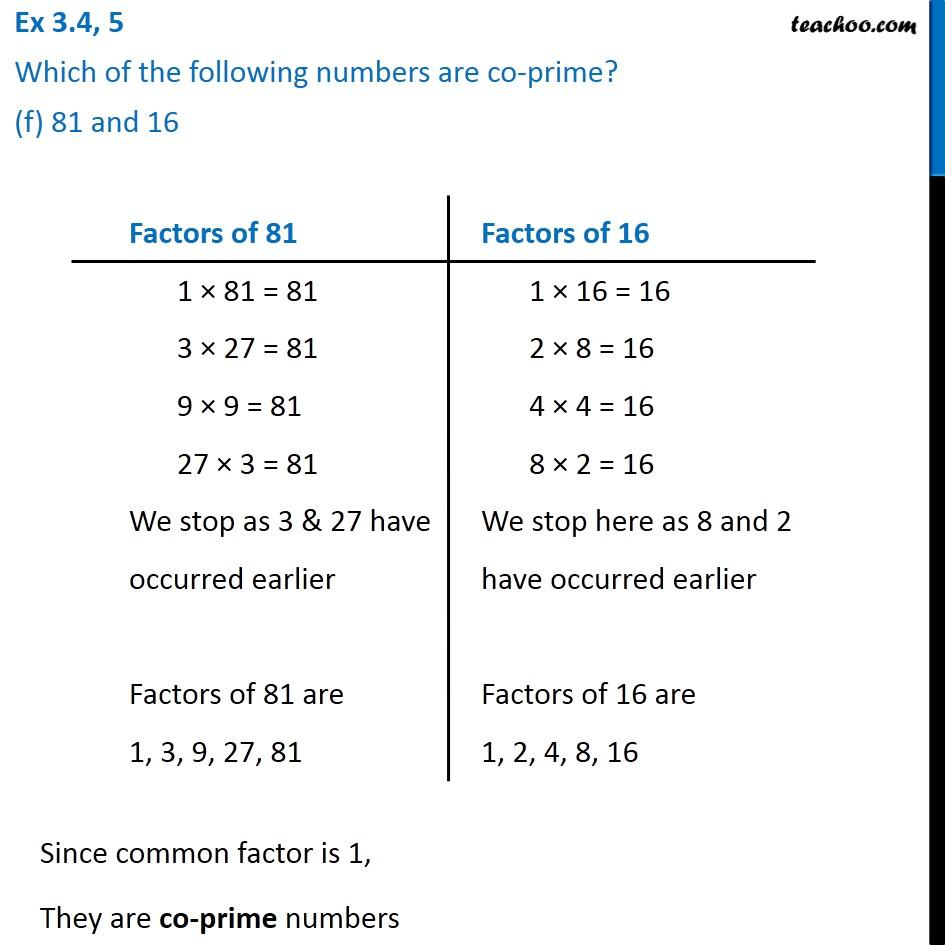Subscribe to our Youtube Channel - https://you.tube/teachoo

1. Chapter 3 Class 6 Playing with Numbers
2. Serial order wise
3. Ex 3.4

Transcript

Ex 3.4, 5 Which of the following numbers are co-prime? (a) 18 and 35 If two Numbers have common factor 1, They are Co-prime Numbers Factors of 18 1 × 18 = 18 2 × 9 = 18 3 × 6 = 18 6 × 3 = 18 We stop here as 3 & 6 have occurred earlier So factors of 18 are 1, 2, 3, 6, 9, and 18 Factors of 35 1 × 35 = 35 5 × 7 = 35 7 × 5 = 35 We stop here as 7 and 5 have occurred earlier So factors of 35 are 1, 5, 7, 35 Since common factor is 1, They are co-prime numbers Ex 3.4, 5 Which of the following numbers are co-prime? (b) 15 and 37 Factors of 15 1 × 15 = 15 3 × 5 = 15 5 × 3 = 15 We stop here as 5 & 3 have occurred earlier So factors of 15 are 1, 3, 5, 15 Factors of 37 1 × 37 = 37 37 × 1 = 37 We stop here as 37 and 1 have occurred earlier So factors of 37 are 1, 37 Since common factor is 1, They are co-prime numbers Ex 3.4, 5 Which of the following numbers are co-prime? (c) 30 and 415 Factors of 30 1 × 30 = 30 2 × 15 = 30 3 × 10 = 30 5 × 6 = 30 6 × 5 = 30 We stop as 6 & 5 have occurred earlier So factors of 30 are 1, 2, 3, 5, 6, 10, 15, 30 Factors of 415 1 × 415 = 415 5 × 83 = 415 83 × 5 = 415 We stop here as 83 and 5 have occurred earlier So factors of 415 are 1, 5, 83, 415 So, common factors are 1, 5 Since common factors are other than 1, They are not co-prime numbers Ex 3.4, 5 Which of the following numbers are co-prime? (d) 17 and 68 Factors of 17 1 × 17 = 17 17 × 1 = 17 We stop as 1 & 17 have occurred earlier So, factors of 17 are 1 and 17 Factors of 68 1 × 68 = 68 2 × 34 = 68 4 × 17 = 68 17 × 4 = 68 We stop as 17 and 4 have occurred earlier So factors of 68 are 1, 2, 4, 17, 34, 68 So, common factors are 1, 17 Since common factors are other than 1, They are not co-prime numbers Ex 3.4, 5 Which of the following numbers are co-prime? (e) 216 and 215 Finding factors of 216 and 215 Factors of 216 1 × 216 = 216 2 × 108 = 216 3 × 72 = 216 4 × 54 = 216 6 × 36 = 216 8 × 27 = 216 9 × 24 = 216 12 × 18 = 216 18 × 12 = 216 We stop as 12 & 18 have occurred earlier Factors of 216 are 1, 2, 3, 4, 6, 8, 9, 12, 18, 22, 27, 36, 54, 72, 108, 216 Factors of 215 1 × 215 = 215 5 × 43 = 215 43 × 5 = 215 We stop here as 5 & 43 have occurred earlier Factors at 215 are 1, 5, 43, 215 Since common factor is 1, They are co-prime numbers Ex 3.4, 5 Which of the following numbers are co-prime? (f) 81 and 16 Factors of 81 1 × 81 = 81 3 × 27 = 17 9 × 9 = 81 27 × 3 = 81 We stop as 3 & 27 have occurred earlier Factors of 81 are 1, 3, 9, 27, 81 Factors of 16 1 × 16 = 16 2 × 8 = 16 4 × 4 = 16 8 × 2 = 16 We stop here as 8 and 2 have occurred earlier Factors of 16 are 1, 2, 4, 8, 16 Factors of 16 1 × 16 = 16 2 × 8 = 16 4 × 4 = 16 8 × 2 = 16 We stop here as 8 and 2 have occurred earlier Factors of 16 are 1, 2, 4, 8, 16 Since common factor is 1, They are co-prime numbers

Ex 3.4